# 3D “Potato” Generation using Sinusoidal Pertubations

Generating unique 3D stimuli can be an art-form.In order to generate “organic” stimuli with smooth undulations, I needed to systematically manipulate the surface meshes of 3D spheres to create smooth peaks and valleys.

To generate 3D “potatoes,” I start with an icosahedron whose mesh is refined to approximate a sphere. The Bioelectromagnetism Matlab Toolbox has a function that is incredibly useful for producing icospheres in MATLAB:

sphere_tri.m requires the following subfunctions (also available in the toolbox):

Calling sphere_tri with no recursive refinement, we get an icosahedron: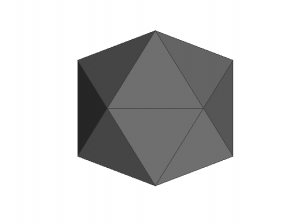With further refinement (increasing nRecurse), we can approximate a sphere. Here’s nRecurse = 1: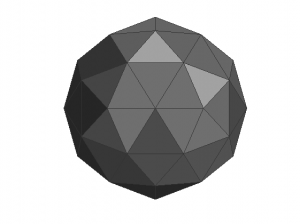nRecurse = 2:nRecurse = 3:nRecurse = 4:nRecurse = 5:I like to use nRecurse = 5 because it produces an icosphere with 10242 vertices (and 20480 faces), which is usually adequate to generate nice-looking “potatoes”. Increasing nRecurse to 6 produces a icosphere with 40962 vertices (and 81920 faces), which is usually more than necessary.

Once we have our icosphere, we want to apply some sinusoidal perturbations to change its shape. The easiest way is to apply a sinusoidal grating to each axis. First let’s define y as vertical, x as horizontal, and z as depth. First, here are some example gratings applied to the X-axis (note that I hold the amplitude of the perturbations constant for these illustrations, but change the angle and frequency):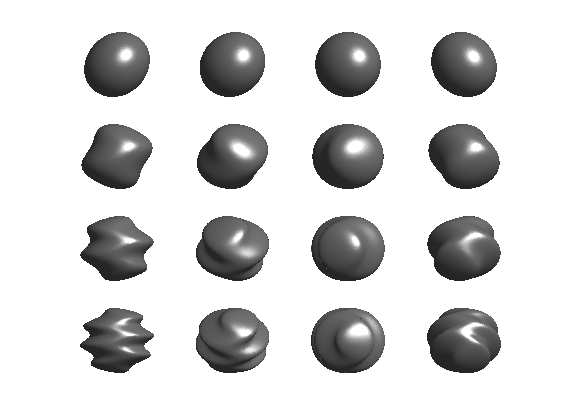Y-axis:And Z-axis:Note that the only thing changing from example to example is the line where the perturbation is applied to the shape’s vertices:

By stacking multiple sinusoidal perturbations, you can produce some nice “blobby potato” stimuli (please excuse the quality of these examples, they were generated using the MATLAB patch command):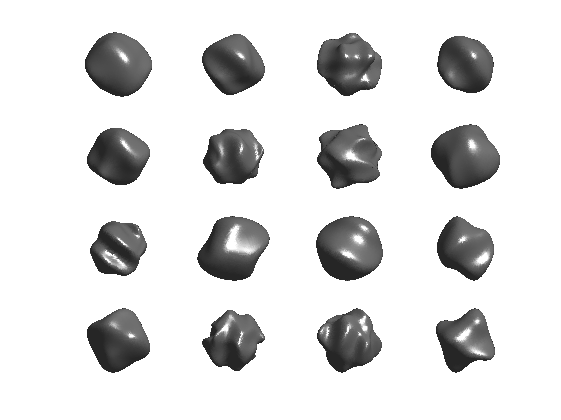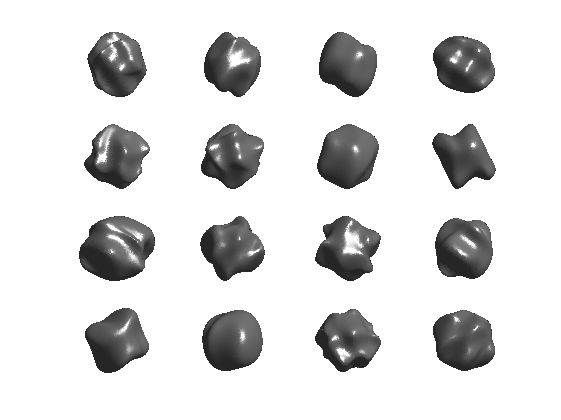## 2 thoughts to “3D “Potato” Generation using Sinusoidal Pertubations”

This site uses Akismet to reduce spam. Learn how your comment data is processed.